Next: The comb function Up: FT AS AN INVERTIBLE Previous: The Nyquist frequency

## Laying out a mesh

In theoretical work and in programs, the definition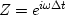is often simplified to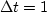,leaving us with.How do we know whetheris given in radians per second or radians per sample? We may not invoke a cosine or an exponential unless the argument has no physical dimensions. So where we seewithout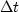,we know it is in units of radians per sample.

In practical work, frequency is typically given in cycles or Hertz, f, rather than radians,(where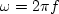). Here we will now switch to f. We will design a computer mesh on a physical object (such as a waveform or a function of space).  We often take the mesh to begin at t=0, and continue till the end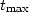of the object, so the time range.Then we decide how many points we want to use. This will be the N used in the discrete Fourier-transform program. Dividing the range by the number gives a mesh interval.

Now let us see what this choice implies in the frequency domain. We customarily take the maximum frequency to be the Nyquist, either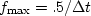Hz or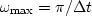radians/sec. The frequency rangegoes fromto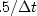.In summary:

•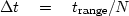is time resolution.
•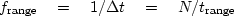is frequency range.
•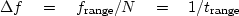is frequency resolution.
In principle, we can always increase N to refine the calculation. Notice that increasing N sharpens the time resolution (makessmaller) but does not sharpen the frequency resolution, which remains fixed. Increasing N increases the frequency range, but not the frequency resolution.

What if we want to increase the frequency resolution? Then we need to choose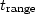larger than required to cover our object of interest. Thus we either record data over a larger range, or we assert that such measurements would be zero. Three equations summarize the facts: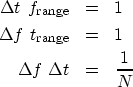(7) (8) (9)

 Increasing range in the time domain increases resolution in the frequency domain and vice versa. Increasing resolution in one domain does not increase resolution in the other.Next: The comb function Up: FT AS AN INVERTIBLE Previous: The Nyquist frequency
Stanford Exploration Project
10/21/1998